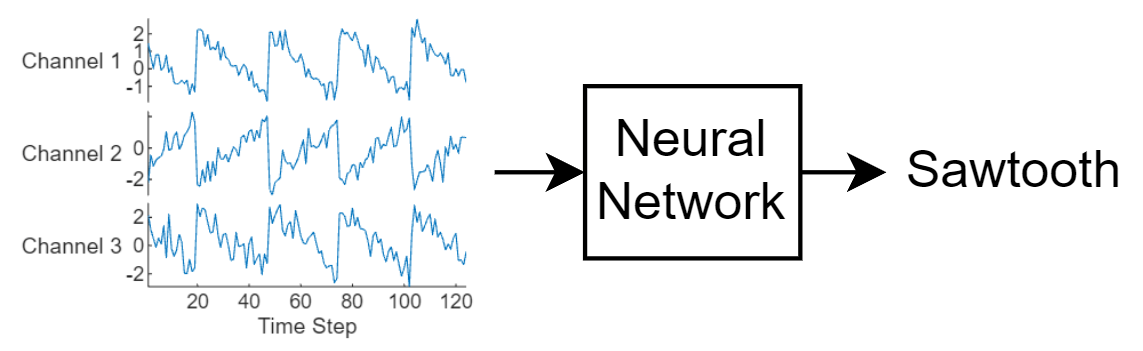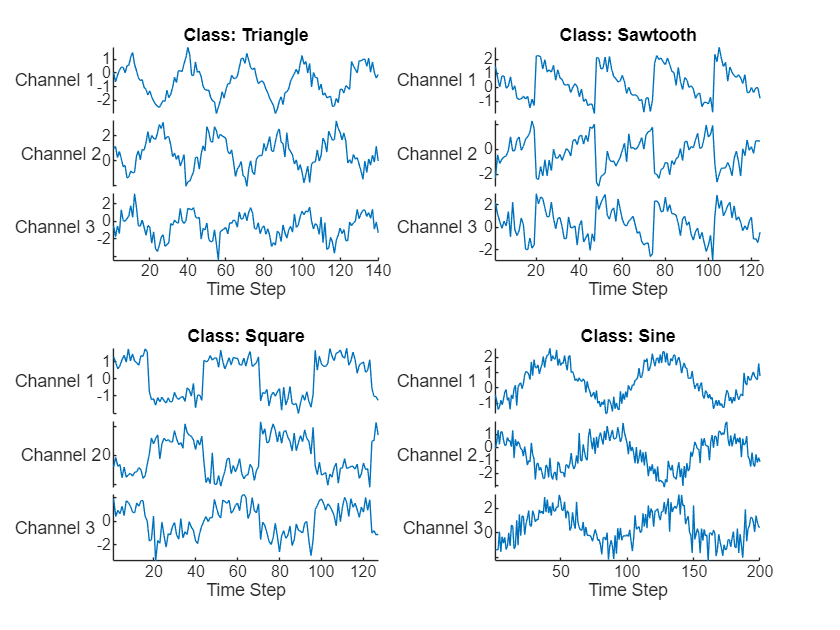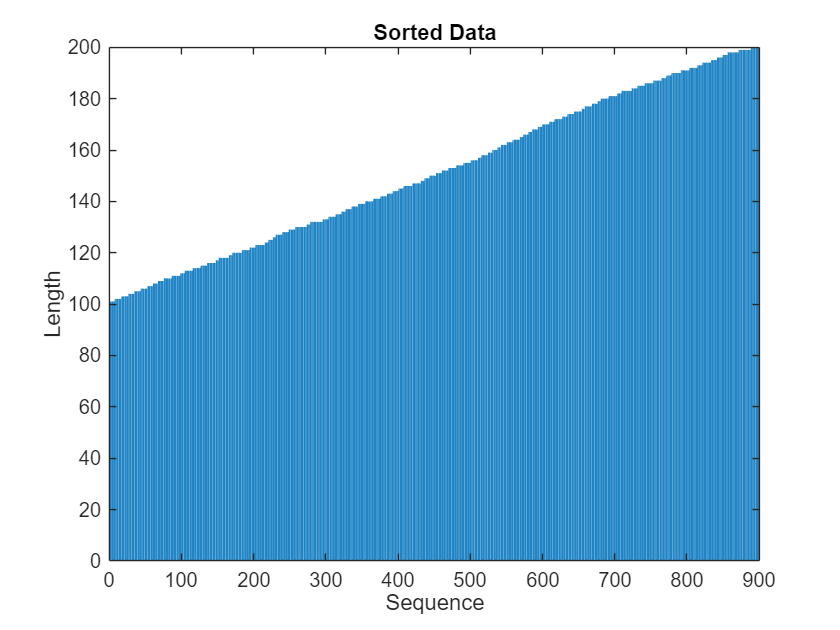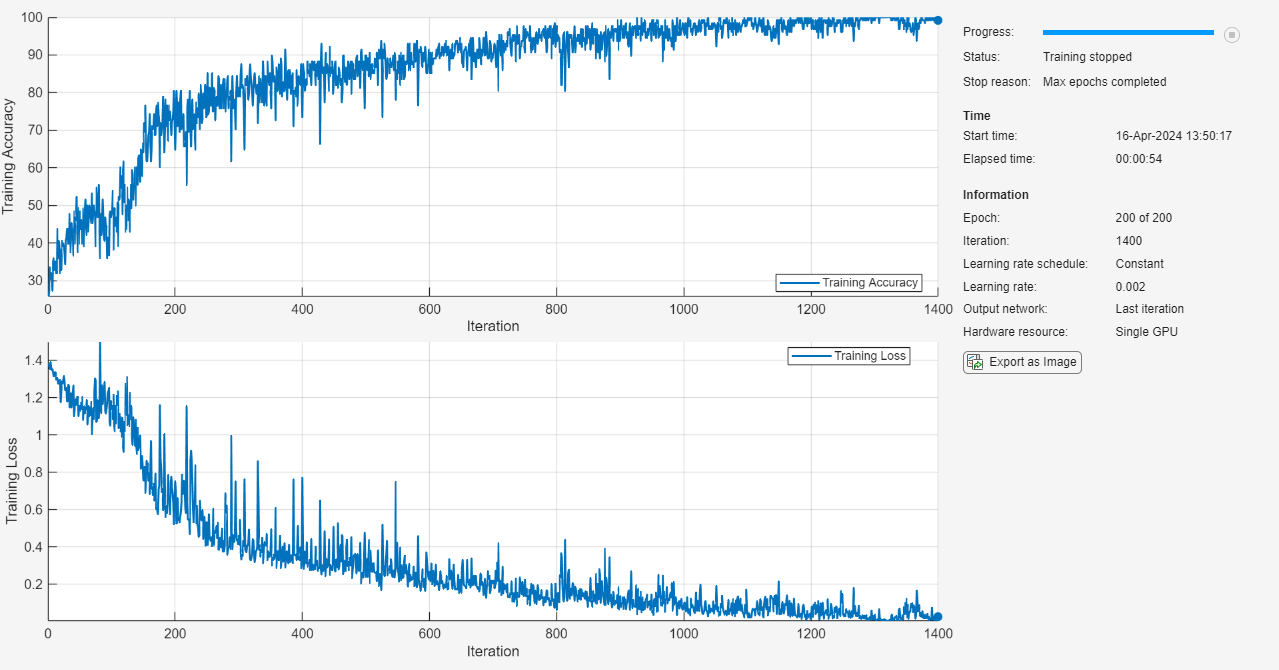# Sequence Classification Using Deep Learning

This example shows how to classify sequence data using a long short-term memory (LSTM) network.

To train a deep neural network to classify sequence data, you can use an LSTM network. An LSTM network enables you to input sequence data into a network, and make predictions based on the individual time steps of the sequence data.

This example uses the Japanese Vowels data set as described in  and . This example trains an LSTM network to recognize the speaker given time series data representing two Japanese vowels spoken in succession. The training data contains time series data for nine speakers. Each sequence has 12 features and varies in length. The data set contains 270 training observations and 370 test observations.

Load the Japanese Vowels training data. `XTrain` is a cell array containing 270 sequences of dimension 12 of varying length. `Y` is a categorical vector of labels "1","2",...,"9", which correspond to the nine speakers. The entries in `XTrain` are matrices with 12 rows (one row for each feature) and varying number of columns (one column for each time step).

```[XTrain,YTrain] = japaneseVowelsTrainData; XTrain(1:5)```
```ans=5×1 cell array {12x20 double} {12x26 double} {12x22 double} {12x20 double} {12x21 double} ```

Visualize the first time series in a plot. Each line corresponds to a feature.

```figure plot(XTrain{1}') xlabel("Time Step") title("Training Observation 1") numFeatures = size(XTrain{1},1); legend("Feature " + string(1:numFeatures),'Location','northeastoutside')```During training, by default, the software splits the training data into mini-batches and pads the sequences so that they have the same length. Too much padding can have a negative impact on the network performance.

To prevent the training process from adding too much padding, you can sort the training data by sequence length, and choose a mini-batch size so that sequences in a mini-batch have a similar length. The following figure shows the effect of padding sequences before and after sorting data.Get the sequence lengths for each observation.

```numObservations = numel(XTrain); for i=1:numObservations sequence = XTrain{i}; sequenceLengths(i) = size(sequence,2); end```

Sort the data by sequence length.

```[sequenceLengths,idx] = sort(sequenceLengths); XTrain = XTrain(idx); YTrain = YTrain(idx);```

View the sorted sequence lengths in a bar chart.

```figure bar(sequenceLengths) ylim([0 30]) xlabel("Sequence") ylabel("Length") title("Sorted Data")```Choose a mini-batch size of 27 to divide the training data evenly and reduce the amount of padding in the mini-batches. The following figure illustrates the padding added to the sequences.

`miniBatchSize = 27;`### Define LSTM Network Architecture

Define the LSTM network architecture. Specify the input size to be sequences of size 12 (the dimension of the input data). Specify an bidirectional LSTM layer with 100 hidden units, and output the last element of the sequence. Finally, specify nine classes by including a fully connected layer of size 9, followed by a softmax layer and a classification layer.

If you have access to full sequences at prediction time, then you can use a bidirectional LSTM layer in your network. A bidirectional LSTM layer learns from the full sequence at each time step. If you do not have access to the full sequence at prediction time, for example, if you are forecasting values or predicting one time step at a time, then use an LSTM layer instead.

```inputSize = 12; numHiddenUnits = 100; numClasses = 9; layers = [ ... sequenceInputLayer(inputSize) bilstmLayer(numHiddenUnits,'OutputMode','last') fullyConnectedLayer(numClasses) softmaxLayer classificationLayer]```
```layers = 5x1 Layer array with layers: 1 '' Sequence Input Sequence input with 12 dimensions 2 '' BiLSTM BiLSTM with 100 hidden units 3 '' Fully Connected 9 fully connected layer 4 '' Softmax softmax 5 '' Classification Output crossentropyex ```

Now, specify the training options. Specify the solver to be `'adam'`, the gradient threshold to be 1, and the maximum number of epochs to be 100. To reduce the amount of padding in the mini-batches, choose a mini-batch size of 27. To pad the data to have the same length as the longest sequences, specify the sequence length to be `'longest'`. To ensure that the data remains sorted by sequence length, specify to never shuffle the data.

Since the mini-batches are small with short sequences, training is better suited for the CPU. Specify `'ExecutionEnvironment'` to be `'cpu'`. To train on a GPU, if available, set `'ExecutionEnvironment'` to `'auto'` (this is the default value).

```maxEpochs = 100; miniBatchSize = 27; options = trainingOptions('adam', ... 'ExecutionEnvironment','cpu', ... 'GradientThreshold',1, ... 'MaxEpochs',maxEpochs, ... 'MiniBatchSize',miniBatchSize, ... 'SequenceLength','longest', ... 'Shuffle','never', ... 'Verbose',0, ... 'Plots','training-progress');```

### Train LSTM Network

Train the LSTM network with the specified training options by using `trainNetwork`.

`net = trainNetwork(XTrain,YTrain,layers,options);`### Test LSTM Network

Load the test set and classify the sequences into speakers.

Load the Japanese Vowels test data. `XTest` is a cell array containing 370 sequences of dimension 12 of varying length. `YTest` is a categorical vector of labels "1","2",..."9", which correspond to the nine speakers.

```[XTest,YTest] = japaneseVowelsTestData; XTest(1:3)```
```ans=3×1 cell array {12x19 double} {12x17 double} {12x19 double} ```

The LSTM network `net` was trained using mini-batches of sequences of similar length. Ensure that the test data is organized in the same way. Sort the test data by sequence length.

```numObservationsTest = numel(XTest); for i=1:numObservationsTest sequence = XTest{i}; sequenceLengthsTest(i) = size(sequence,2); end [sequenceLengthsTest,idx] = sort(sequenceLengthsTest); XTest = XTest(idx); YTest = YTest(idx);```

Classify the test data. To reduce the amount of padding introduced by the classification process, set the mini-batch size to 27. To apply the same padding as the training data, specify the sequence length to be `'longest'`.

```miniBatchSize = 27; YPred = classify(net,XTest, ... 'MiniBatchSize',miniBatchSize, ... 'SequenceLength','longest');```

Calculate the classification accuracy of the predictions.

`acc = sum(YPred == YTest)./numel(YTest)`
```acc = 0.9730 ```

 M. Kudo, J. Toyama, and M. Shimbo. "Multidimensional Curve Classification Using Passing-Through Regions." Pattern Recognition Letters. Vol. 20, No. 11–13, pages 1103–1111.

 UCI Machine Learning Repository: Japanese Vowels Dataset. https://archive.ics.uci.edu/ml/datasets/Japanese+Vowels